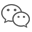## Corn Fields（状压DP）

Corn Fields
 Time Limit: 2000MS Memory Limit: 65536K Total Submissions: 23437 Accepted: 12275

Description

Farmer John has purchased a lush new rectangular pasture composed of M by N (1 ≤ M ≤ 12; 1 ≤ N ≤ 12) square parcels. He wants to grow some yummy corn for the cows on a number of squares. Regrettably, some of the squares are infertile and can't be planted. Canny FJ knows that the cows dislike eating close to each other, so when choosing which squares to plant, he avoids choosing squares that are adjacent; no two chosen squares share an edge. He has not yet made the final choice as to which squares to plant.

Being a very open-minded man, Farmer John wants to consider all possible options for how to choose the squares for planting. He is so open-minded that he considers choosing no squares as a valid option! Please help Farmer John determine the number of ways he can choose the squares to plant.

Input

Line 1: Two space-separated integers: M and N
Lines 2..M+1: Line i+1 describes row i of the pasture with N space-separated integers indicating whether a square is fertile (1 for fertile, 0 for infertile)

Output

Line 1: One integer: the number of ways that FJ can choose the squares modulo 100,000,000.

Sample Input

```2 3
1 1 1
0 1 0```

Sample Output

`9`

Hint

Number the squares as follows:
`1 2 3  4  `

There are four ways to plant only on one squares (1, 2, 3, or 4), three ways to plant on two squares (13, 14, or 34), 1 way to plant on three squares (134), and one way to plant on no squares. 4+3+1+1=9.``` 1 #include <iostream>
2 #include <cstring>
3 #include <cstdio>
4 #include <algorithm>
5
6 using namespace std;
7 int n,m;
8 const int maxn=13;
9 const int mod=1e8;
10 int arr;
11 int state[1<<maxn];
12 int ee[maxn];   //每一行的状态
13 int dp[maxn][1<<maxn];  //第i行采用第j种状态的方案数量
14
15
16 int main(){
17     ios::sync_with_stdio(false);
18     dp=1;  //全部不填为1
19     cin>>n>>m;
20     for(int i=1;i<=n;i++){
21         for(int j=1;j<=m;j++){
22             cin>>arr[i][j];
23         }
24     }
25     int len=1<<m;
26     for(int i=0;i<len;i++){   //每一种状态自己会不会行冲突
27         state[i]=((i&(i<<1))==0&&(i&(i>>1))==0); //1表示不冲突,0表示冲突
28     }
29     for(int i=1;i<=n;i++){
30         for(int j=1;j<=m;j++){
31             ee[i]=(ee[i]<<1)|arr[i][j];   //每一行的状态
32         }
33     }
34     for(int i=1;i<=n;i++){
35         for(int j=0;j<len;j++){
36             if(state[j]&&(j&ee[i])==j){   //首先不冲突其次状态为i行的状态
37                 for(int k=0;k<len;k++){
38                     if((k&j)==0){   //这一行与上一行的 列没有冲突
39                         dp[i][j]=(dp[i][j]+dp[i-1][k])%mod;   //不要忘记取余
40                     }
41                 }
42             }
43         }
44     }
45     int res=0;
46     for(int i=0;i<len;i++){
47         res+=dp[n][i];
48         res%=mod;   //不要忘记取余
49     }
50     cout << res << endl;
51     return 0;
52 }```
View Code

##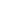咨询·合作

•### 售前咨询

•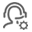### 售后服务

•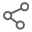### 渠道合作Tel：15866163259

欢迎各方咨询洽谈、业务合作及项目开发

•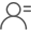### 大客户合作Tel：15866163259

可提供产品定制服务

•